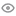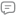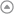New update is available. Click here to update.-> Rishabh Jain, a passionate B.Tech student specializing in Computer Science at College of Engineering Roorkee. With a love for coding, he aspires to become a coding ninja. Mastering various programm...College of Engineering Roorkee 2025My StatsEXP gained19061Level
7 (Expert)
Community statsDiscussions
25
Know more759
Total problems solved
744
Easy
15
Moderate
0
Hard
0
NinjaCurrent streak:

0 days

Longest streak:

24 days

Less

More

Achievements4
Ronin
Topics
Arrays
Sorting
Strings
+ 1 more1
Ronin
Guided path
Basics of C++2
Sensei
Guided path
Pointers
ChatGPT1 Time topper

College of Engineering Roorkee291 average partcipants
Discussions
Five simple approaches 🙌||Easiest CPP solution🚀
Interview problems

// we can swap by taking a third variable

void swapNumber(int &a, int &b) {

int temp=0;

temp=a;

a=b;

b=temp;

}

// we can swap with our inbuilt swap function

void swapNumber(int &a, int &b) {

swap(a,b);

}

//using + and - operator

void swapNumber(int &a, int &b){

a=a+b;

b=a-b;

a=a-b;

}

//using * and / operator

void swapNumber(int &a, int &b){

a=a*b;

b=a/b;

a=a/b;

}

//using xor ^ operator

void swapNumber(int &a, int &b){

a=a^b;

b=a^b;

a=a^b;

}

//Kindly upvote if this helps you in any way.

Enrish
Published On 30-Oct-2023128 views0 repliesFive simple approaches 🙌||Easiest CPP solution🚀
Interview problems

// we can swap by taking a third variable

void swapNumber(int &a, int &b) {

int temp=0;

temp=a;

a=b;

b=temp;

}

// we can swap with our inbuilt swap function

void swapNumber(int &a, int &b) {

swap(a,b);

}

//using + and - operator

void swapNumber(int &a, int &b){

a=a+b;

b=a-b;

a=a-b;

}

//using * and / operator

void swapNumber(int &a, int &b){

a=a*b;

b=a/b;

a=a/b;

}

//using xor ^ operator

void swapNumber(int &a, int &b){

a=a^b;

b=a^b;

a=a^b;

}

//Kindly upvote if this helps you in any way.

Enrish
Published On 30-Oct-2023128 views0 repliesFive simple approaches 🙌||Easiest CPP solution🚀
Interview problems

// we can swap by taking a third variable

void swapNumber(int &a, int &b) {

int temp=0;

temp=a;

a=b;

b=temp;

}

// we can swap with our inbuilt swap function

void swapNumber(int &a, int &b) {

swap(a,b);

}

//using + and - operator

void swapNumber(int &a, int &b){

a=a+b;

b=a-b;

a=a-b;

}

//using * and / operator

void swapNumber(int &a, int &b){

a=a*b;

b=a/b;

a=a/b;

}

//using xor ^ operator

void swapNumber(int &a, int &b){

a=a^b;

b=a^b;

a=a^b;

}

//Kindly upvote if this helps you in any way.

Enrish
Published On 30-Oct-2023128 views0 repliesFive simple approaches 🙌||Easiest CPP solution🚀
Interview problems

// we can swap by taking a third variable

void swapNumber(int &a, int &b) {

int temp=0;

temp=a;

a=b;

b=temp;

}

// we can swap with our inbuilt swap function

void swapNumber(int &a, int &b) {

swap(a,b);

}

//using + and - operator

void swapNumber(int &a, int &b){

a=a+b;

b=a-b;

a=a-b;

}

//using * and / operator

void swapNumber(int &a, int &b){

a=a*b;

b=a/b;

a=a/b;

}

//using xor ^ operator

void swapNumber(int &a, int &b){

a=a^b;

b=a^b;

a=a^b;

}

//Kindly upvote if this helps you in any way.

Enrish
Published On 30-Oct-2023128 views0 repliesFive simple approaches 🙌||Easiest CPP solution🚀
Interview problems

// we can swap by taking a third variable

void swapNumber(int &a, int &b) {

int temp=0;

temp=a;

a=b;

b=temp;

}

// we can swap with our inbuilt swap function

void swapNumber(int &a, int &b) {

swap(a,b);

}

//using + and - operator

void swapNumber(int &a, int &b){

a=a+b;

b=a-b;

a=a-b;

}

//using * and / operator

void swapNumber(int &a, int &b){

a=a*b;

b=a/b;

a=a/b;

}

//using xor ^ operator

void swapNumber(int &a, int &b){

a=a^b;

b=a^b;

a=a^b;

}

//Kindly upvote if this helps you in any way.

Enrish
Published On 30-Oct-2023128 views0 repliesDutch national Flag Algo 🚀with explaination||Easiest CPP solution 🙌||
Interview problems

dutch nationial flag algo basically deals with the thing that 0 is in beginning , 1 in mid and 2 in the end, you can see that easily in below code.

#include <bits/stdc++.h>

void sort012(int *arr, int n)

{

int low=0,high=n-1,mid=0;

while(high>=mid)

{

if(arr[mid]==0)

{

swap(arr[low],arr[mid]);

low++;

mid++;

}

else if(arr[mid]==1)

{

mid++;

}

else if(arr[mid]==2)

{

swap(arr[mid],arr[high]);

high--;

}

}

}

Kindly upvote if it helps you in anyway :)

Enrish
Published On 30-Oct-202398 views0 repliesDutch national Flag Algo 🚀with explaination||Easiest CPP solution 🙌||
Interview problems

dutch nationial flag algo basically deals with the thing that 0 is in beginning , 1 in mid and 2 in the end, you can see that easily in below code.

#include <bits/stdc++.h>

void sort012(int *arr, int n)

{

int low=0,high=n-1,mid=0;

while(high>=mid)

{

if(arr[mid]==0)

{

swap(arr[low],arr[mid]);

low++;

mid++;

}

else if(arr[mid]==1)

{

mid++;

}

else if(arr[mid]==2)

{

swap(arr[mid],arr[high]);

high--;

}

}

}

Kindly upvote if it helps you in anyway :)

Enrish
Published On 30-Oct-202398 views0 repliesDutch national Flag Algo 🚀with explaination||Easiest CPP solution 🙌||
Interview problems

dutch nationial flag algo basically deals with the thing that 0 is in beginning , 1 in mid and 2 in the end, you can see that easily in below code.

#include <bits/stdc++.h>

void sort012(int *arr, int n)

{

int low=0,high=n-1,mid=0;

while(high>=mid)

{

if(arr[mid]==0)

{

swap(arr[low],arr[mid]);

low++;

mid++;

}

else if(arr[mid]==1)

{

mid++;

}

else if(arr[mid]==2)

{

swap(arr[mid],arr[high]);

high--;

}

}

}

Kindly upvote if it helps you in anyway :)

Enrish
Published On 30-Oct-202398 views0 repliesDutch national Flag Algo 🚀with explaination||Easiest CPP solution 🙌||
Interview problems

dutch nationial flag algo basically deals with the thing that 0 is in beginning , 1 in mid and 2 in the end, you can see that easily in below code.

#include <bits/stdc++.h>

void sort012(int *arr, int n)

{

int low=0,high=n-1,mid=0;

while(high>=mid)

{

if(arr[mid]==0)

{

swap(arr[low],arr[mid]);

low++;

mid++;

}

else if(arr[mid]==1)

{

mid++;

}

else if(arr[mid]==2)

{

swap(arr[mid],arr[high]);

high--;

}

}

}

Kindly upvote if it helps you in anyway :)

Enrish
Published On 30-Oct-202398 views0 repliesSmallest || Optimized CPP sol 🧑‍💻|| Very easy approach🚀🙌
Interview problems

#include <iostream>

using namespace std;

int main() {

int Length;

return 0;

}

Enrish
Published On 30-Oct-2023148 views0 replies